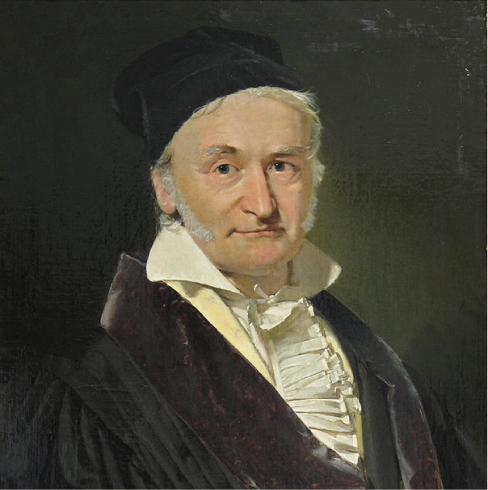Gauss’s Law

# 36 Introduction

This chapter introduces the concept of flux, which relates a physical quantity and the area through which it is flowing. Although we introduce this concept with the electric field, the concept may be used for many other quantities, such as fluid flow. (credit: modification of work by “Alessandro”/Flickr)Flux is a general and broadly applicable concept in physics. However, in this chapter, we concentrate on the flux of the electric field. This allows us to introduce Gauss’s law, which is particularly useful for finding the electric fields of charge distributions exhibiting spatial symmetry. The main topics discussed here are

1. Electric flux. We define electric flux for both open and closed surfaces.
2. Gauss’s law. We derive Gauss’s law for an arbitrary charge distribution and examine the role of electric flux in Gauss’s law.
3. Calculating electric fields with Gauss’s law. The main focus of this chapter is to explain how to use Gauss’s law to find the electric fields of spatially symmetrical charge distributions. We discuss the importance of choosing a Gaussian surface and provide examples involving the applications of Gauss’s law.
4. Electric fields in conductors. Gauss’s law provides useful insight into the absence of electric fields in conducting materials.

So far, we have found that the electrostatic field begins and ends at point charges and that the field of a point charge varies inversely with the square of the distance from that charge. These characteristics of the electrostatic field lead to an important mathematical relationship known as Gauss’s law. This law is named in honor of the extraordinary German mathematician and scientist Karl Friedrich Gauss ((Figure)). Gauss’s law gives us an elegantly simple way of finding the electric field, and, as you will see, it can be much easier to use than the integration method described in the previous chapter. However, there is a catch—Gauss’s law has a limitation in that, while always true, it can be readily applied only for charge distributions with certain symmetries.

Karl Friedrich Gauss (1777–1855) was a legendary mathematician of the nineteenth century. Although his major contributions were to the field of mathematics, he also did important work in physics and astronomy.